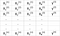# Introduction

In this article, we will consider three examples of real and symmetric matrix models that we often encounter in data science and machine learning, namely, the regression matrix (R); the covariance matrix, and the linear discriminant analysis matrix (L).

# Example 1: Linear Regression MatrixTable 1. Features matrix with 4 variables and n observations. Column 5 is the target variable (y).

We would like to build a multi-regression model for predicting the y values (column 5). Our model can thus be expressed in the form

In matrix form, this equation can be written as

where X is the ( n x 4) features matrix, w is the (4 x 1) matrix representing the regression coefficients to be determined, and y is the (n x 1) matrix containing the n observations of the target variable y.

Note that X is a rectangular matrix, so we can’t solve the equation above by taking the inverse of X.

To convert X into a square matrix, we multiple the left-hand side and right-hand side of our equation by the transpose of X, that is

This equation can also be expressed as

where

is the (4 x 4) regression matrix. Clearly, we observe that R is a real and symmetric matrix. Note that in linear algebra, the transpose of the product of two matrices obeys the following relationship

Now that we’ve reduced our regression problem and expressed it in terms of the (4x4) real, symmetric, and invertible regression matrix R, it is straightforward to show that the exact solution of the regression equation is then

# Example 2: Covariance Matrix

To visualize the correlations between the features, we can generate a scatter plot. To quantify the degree of correlation between features (multicollinearity), we can compute the covariance matrix using this equation:

In matrix form, the covariance matrix can be expressed as a 4 x 4 real and symmetric matrix:

Again, we see that the covariant matrix is real and symmetric. This matrix can be diagonalized by performing a unitary transformation, also referred to as Principal Component Analysis (PCA) transformation to obtain the following:

Since the trace of a matrix remains invariant under a unitary transformation, we observe that the sum of the eigenvalues of the diagonal matrix is equal to the total variance contained in features X1, X2, X3, and X4.

# Example 3: Linear Discriminant Analysis Matrix

where S_W is the within-feature scatter matrix, and S_B is the between-feature scatter matrix. Since both matrices S_W and S_B are real and symmetric, it follows that L is also real and symmetric. The diagonalization of L produces a feature subspace that optimizes class separability and reduces dimensionality. Hence LDA is a supervised algorithm, while PCA is not.

For more details about the implementation of LDA, please see the following references:

Machine Learning: Dimensionality Reduction via Linear Discriminant Analysis

GitHub repository for LDA implementation using Iris dataset

Python Machine Learning by Sebastian Raschka, 3rd Edition (Chapter 5)

# Additional Data Science/Machine Learning Resources

Data Science Curriculum

5 Best Degrees for Getting into Data Science

Theoretical Foundations of Data Science — Should I Care or Simply Focus on Hands-on Skills?

Machine Learning Project Planning

How to Organize Your Data Science Project

Productivity Tools for Large-scale Data Science Projects

A Data Science Portfolio is More Valuable than a Resume

For questions and inquiries, please email me: benjaminobi@gmail.com

Written by

Written by

## Benjamin Obi Tayo Ph.D.

#### Physicist, Data Science Educator, Writer. Interests: Data Science, Machine Learning, AI, Python & R, Predictive Analytics, Materials Sciences, Biophysics